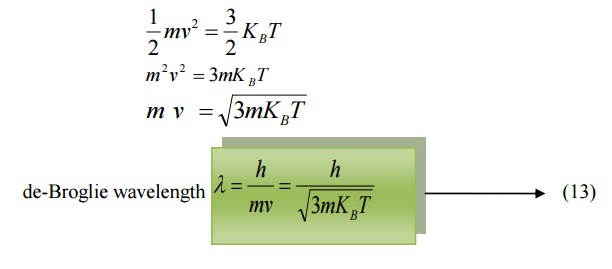Home | | Engineering Physics I | Dual Nature of Radiation (Light) And Matter (Particles) - Matter Waves

# Dual Nature of Radiation (Light) And Matter (Particles) - Matter Waves

de- Broglie concept of dual nature: The universe is made of radiation (light) and matter (particles). The light exhibits the dual nature (ie.) it can behave both a wave (interference, diffraction, phenomenon) and as a particle (Compton Effect, photo electric effect etc.)

DUAL NATURE OF RADIATION (LIGHT) AND MATTER (PARTICLES) – MATTER WAVES

de- Broglie concept of dual nature:

The universe is made of radiation (light) and matter (particles). The light exhibits the dual nature (ie.) it can behave both a wave (interference, diffraction, phenomenon) and as a particle (Compton Effect, photo electric effect etc.)

Since the nature loves symmetry, in 1923 Louis debroglie suggested that an electron or any other material particle must exhibit wave like properties in addition to

particle nature.

The waves associated with a material particle are called as matter waves. De-Broglie wavelength:

From the theory of light, considering a photon as a particle the total energy of the photon is given byWhere m is the mass of the particle

c is the velocity of light

Considering the photon as a wave, the total energy is given by

E = hγ ------------- > (2)

Where h is the Planck’s constant

Γ is the frequency of the radiation.

From equations (1) and (2)

E = mc2 = hγ    --------- ((3))

We know Momentum = Mass × velocity

p = mc

hv=pcde-Broglie suggested that the equation 3 can be applied both for photons and material particles. If m is the mass of the particle and v is the velocity the particle, then

Momentum p = mv6.1 de-Broglie wavelength in terms of energy6.2 de-Broglie wavelength in terms of Voltage

If a charged particle of charge ’e’ is accelerated through a potential difference

Then the Kinetic Energy of the particle = ½ mv2  --à (7)

Also we know energy = eV           -------- (8)

Equating 7 and 8, we get,Multiplying by ‘m’ both sides, we getSubstituting 9 in 5, we get

de-Broglie wavelength6.3 de-Broglie wavelength in terms of Temperature

When a particle like neutron is in thermal equilibrium at temperature T, then they possess Maxwell distribution of velocities.Where vrm s  is the Root mean square velocity of the particle.

Also, we know energy =Where KB  is the Boltzmann constant.

Equating (11) and (12) we getStudy Material, Lecturing Notes, Assignment, Reference, Wiki description explanation, brief detail
Physics : Quantum Physics : Dual Nature of Radiation (Light) And Matter (Particles) - Matter Waves |